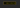# How to use es6 Spread(...) operator JavaScriptThe spread syntax allows us to expand the arrays or strings where zero or more arguments are needed for the function calls or array literals or object literals.

Let’s see in practice

### Combine arrays

``````const fruits = ['apples', 'apricots', 'avocados'];

const veg = ['broccoli','corn','cucumber'];

const vegplusfruits = [...fruits,...veg];

console.log(vegplusfruits)

//output

In the above code, we combined two arrays into a single array by using the spread operator.

### Function calls

Consider we have an array of numbers we need to pass into the function as an arguments.

``````function sum (a,b,c,d,e,f,g,h){

return a+b+c+d+e+f+g+h;

}

var numbers =  [1,2,3,4,5,6,7,8];

console.log(sum(...numbers));

//output -> 36``````

### Copy Objects

``````const user1 = {
id: 1,
name: "king",
}

const copyUser1 = {
...user1
}

console.log(copyUser1)

/*
output

{
id: 1,
name: "king"
}

*/``````

### Strings

We can also split the single string into an array of strings by using the spread operator.

``````let name = "JavaScript"

console.log([...name]);

// ["J", "a", "v", "a", "S", "c", "r", "i", "p", "t"]``````

Happy coding…